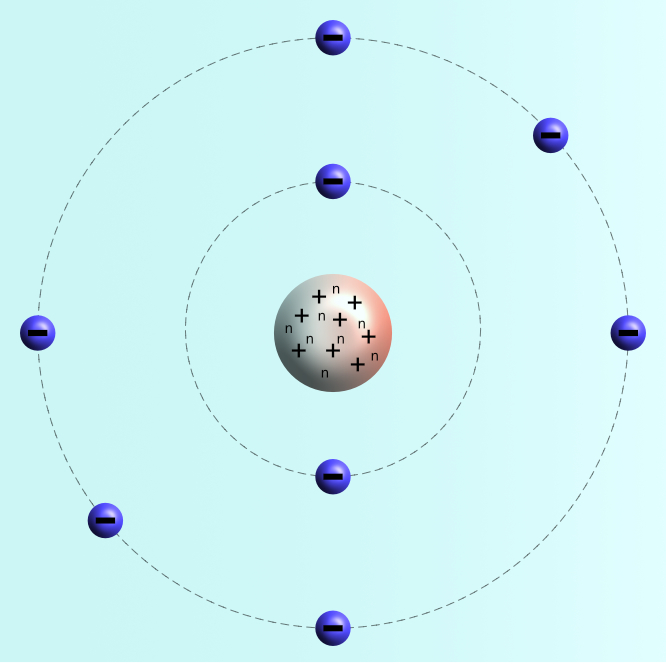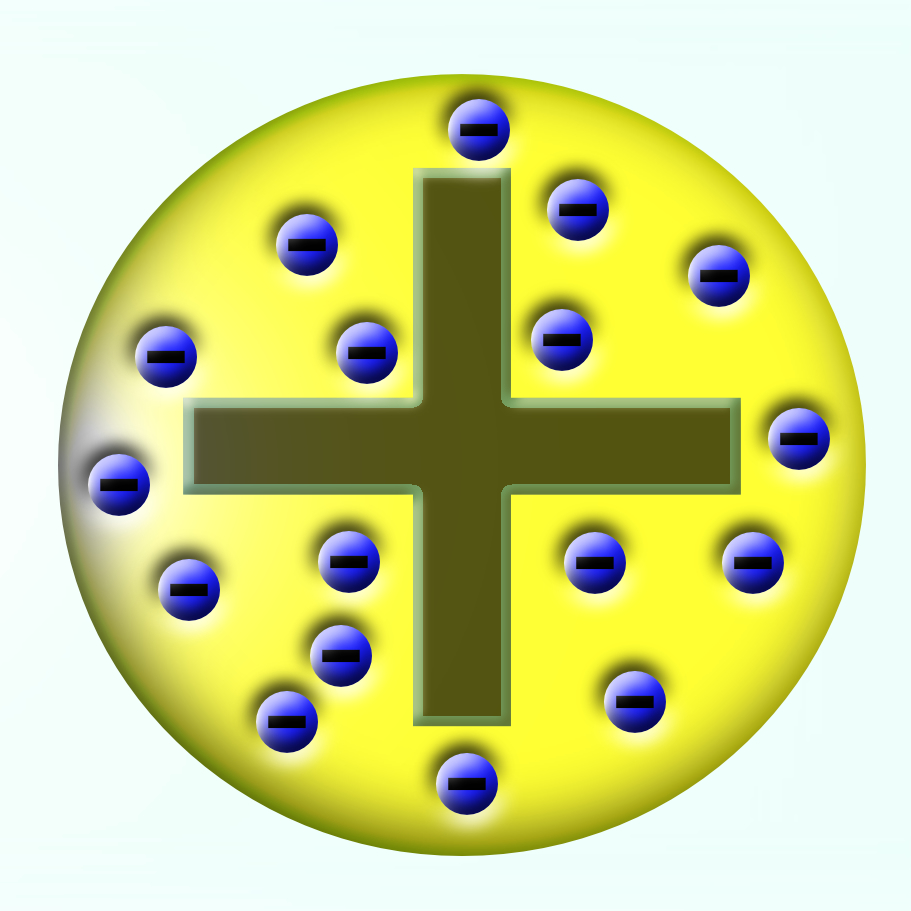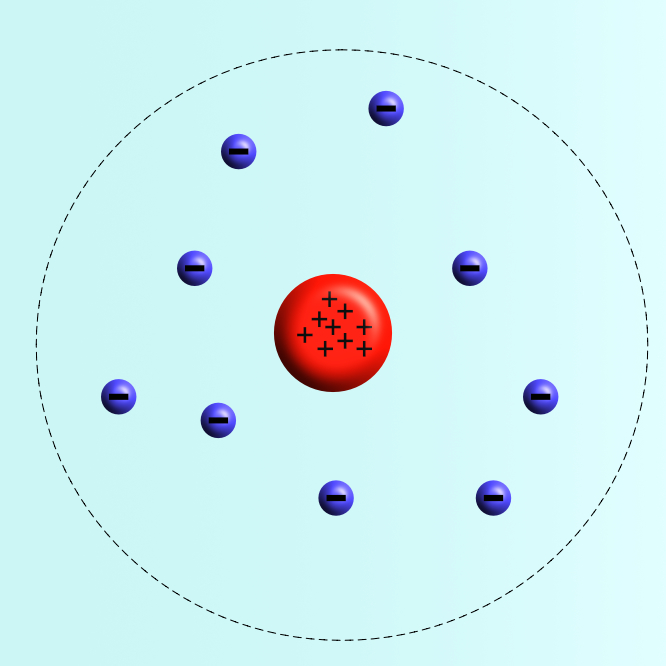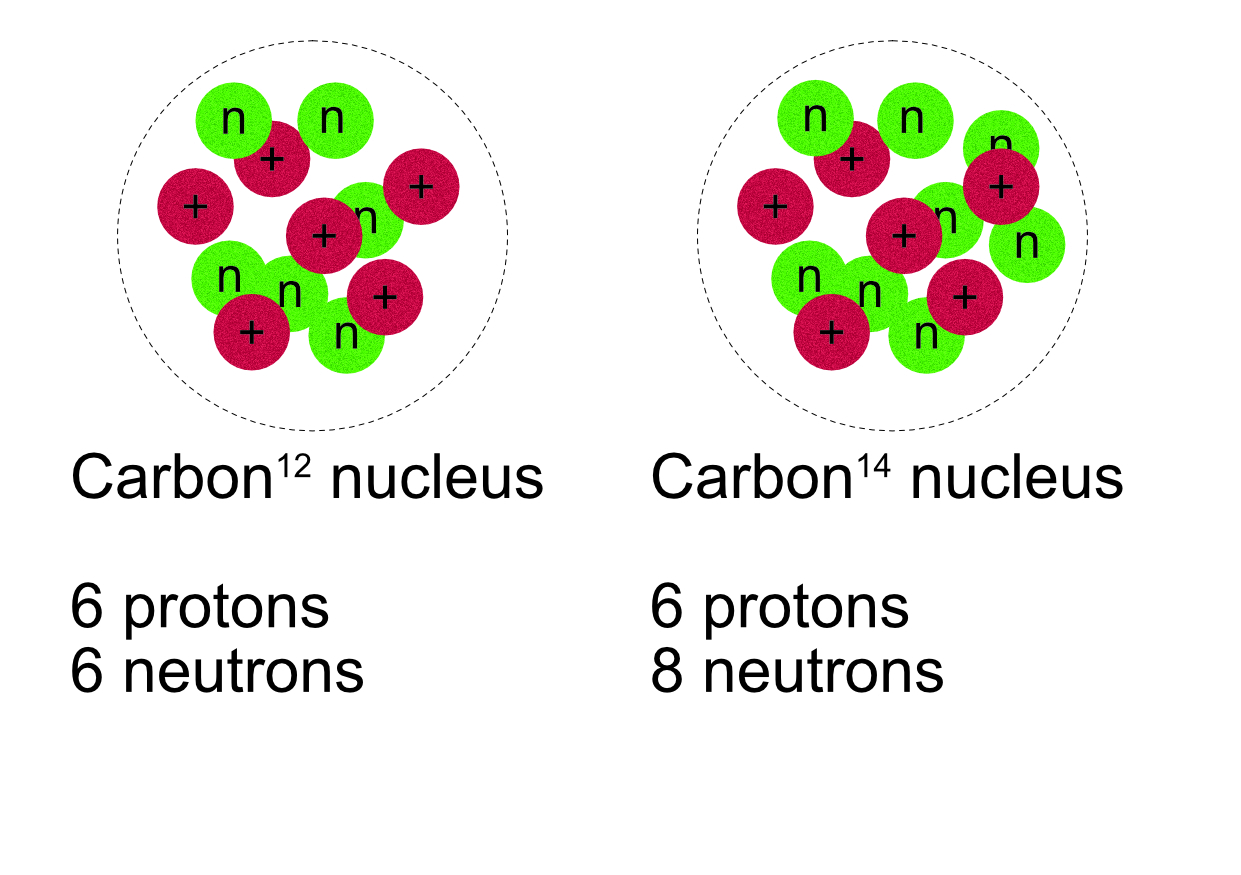# Atomic Structure

## Atomic Structure

Atoms are the smallest unit of chemistry that have the properties of an individual element. Atoms are made of three smaller, sub-atomic, particles.

1. Electrons
2. Protons
3. Neutrons

Properties of sub-atomic particles.

 Particle Mass (atomic mass) Charge Location in atom Electron 0 -1 Orbiting nucleus Proton 1 +1 nucleus Neutron 1 none nucleus

The standard physical model of an atom is based on the work of Niels Bohr, a Danish scientist.Example: Oxygen (168O)

Atomic mass 16, (number of protons + neutrons).

Atomic number 8, (number of protons).

8 neutrons (n) and 8 protons (+) are found in the centre of the atom called the nucleus. This contains all the mass of the atom and is positively charged.

The 8 positive protons in the nucleus are balanced by 8 negative electrons orbiting the nucleus.

Electrons are found in orbits around the nucleus, each orbit can hold a maximum number of electrons before it is full.

1st orbit : 2 electrons

2nd orbit : 8 electrons

3rd orbit : 8 electrons.

Note: For the GCSE examination only the number of electrons in the first three orbits are required.

The nucleus is very small compared to the total radius of the whole atom, but it contains almost all the mass of the atom. Atoms are very small, an oxygen atom, for example, is only 6 x 10-11 m across. Most simple compounds are no larger than 10-10 m in diameter.

Calculating the number of sub-atomic particles inan atom.

Number of Protons = atomic number

Number of electrons = number of protons.

Number of neutrons = atomic mass - atomic number

Example

Elements are listed in the periodic table using their atomic symbol and with two numbers. The atomic mass which is the number of particles in the nucleus and the atomic number which is the number of protons in the nucleus.

Atomic mass Atomic number X Oxygen is 168O

Protons = atomic number = 8

Electrons = protons = 8

Neutrons = atomic mass - atomic number = 16 - 8 = 8

Example 2

Neon 2010Ne atomic mass = 20 atomic number = 10

Protons = atomic number = 10

Electrons = protons = 10

Neutrons = atomic mass - atomic number = 10

## Ions

All atoms are electrically neutral, this means that they have the same number of protons and electrons, equal numbers of positive and negative particles. An ion is an electrically charged atoms, this means that it has either gained electrons or lost them.

1. Gaining electrons makes an atom negatively charged.
2. Losing electrons makes the atom positively charged.

Example 1

Sodium atom 2311Na Protons = 11, electrons = 11, neutrons = 23 -11 = 12

Sodium ion 2311Na+ Protons = 11, electrons = 10, neutrons = 12

For an ion, the number of proton and neutrons does not change. The “+” indicates there is 1 more proton than electrons. “+2” would mean 2 more protons than electrons etc. A “-” indicates thenumber of additional electrons.

Example 2

Magnesium atom 2412Mg Protons = 12, electrons = 12, neutrons = 24 -12 = 12

Magnesium ion 2412Mg+2 Protons = 12, electrons = 10, neutrons = 12

Example 3

Fluorine atom 199F Protons = 9, electrons = 9, neutrons = 19 -9 =10

Fluorine ion 199F- Protons =9, electrons =10, neutrons = 10

1. Metal elements lose electrons to become positive ions.
2. Non-metal elements gain electrons to become negative ions.

## Development of the Model

The idea of an atom as the basic unit of matter was first suggested by Democritus in ancient Greece around 400 BC. He suggested that the atom was the smallest unit of matter and could not be divided into anything simpler. (Atom comes from the Greek word Atomos - not to divide).

This idea remained as the basic model of matter until 1803 when John Dalton adapted the idea and suggested that elements were atoms of different sizes. He still considered them as solid objects that could not be divided.

In 1897, JJ Thomson discovered the electron and realised that they came from within the atom. As he knew that all atoms are electrically neutral and the electron was negative he suggested that the atom must be a “lump” of positive material with the negative electrons embedded in it.JJ Thomson’s 1904 model of the atom became known as the plum pudding model.

A year later in 1905, Ernest Rutherford, working with two of his students Marsden and Geiger, tested this model by firing positively charged alpha radiation particles at a thin leaf of gold to see how they reacted. They noticed that some were deflected by large angles, suggesting that the positive part of the atom was concentrated in the middle.Rutherford’s 1905 model placed the protons in the middle with a cloud of electrons around the outside. He also realized that the nucleus was very small compared to the overall size of the atoms.

In 1913, Niels Bohr adapted this model and placed the electrons in orbits around the nucleus. He studied the way atoms behaved when they absorb and emit electromagnetic radiation to support his theory. This is still the model used today, with the addition of the neutrons in the nucleus.

## Isotopes

The mass of an atom depends upon the total number of sub-atomic particles in its nucleus, (protons + neutrons). The type of element of an atom depends upon the number of protons. Changing the number of neutrons will change the overall mass of the atom, it also affects its stability - see notes on physics for this course for more details.

Atoms with the same number of protons, (same element), but with a different number of neutrons are called isotopes of an element, (different atomic masses).These two nuclei are both types of carbon, Carbon-12 and Carbon-14. They are both isotopes of carbon. Carbon-14 has two more neutrons than the Carbon-12 isotope. They have the same number of protons and the same number of electrons.

Carbon-12 126C protons = 6, electrons = 6, neutrons = 12 -6 = 6

Carbon-14 146C protons = 6, electrons = 6, neutrons = 14 -6 = 8

A particle can be both an isotope of an element and an ion. This will change the number of electrons and the number of neutrons, but not the number of protons.